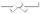# Exercises

0 / 50

For this question, assume the following implementation of the Person and Student classes:

#### Person Class Implementation

``public class Person...``0 / 50

For the question below, assume the following implementation of the Measurable interface and Square and Circle classes:

``public class Shape...``0 / 50

Write a `LinkedBag<T>` member method called `contains()` that takes an element as a parameter and returns true if the bag contains the...0 / 50

For this exercise, you are writing code in the following implementation of ArrayBag:

``public static final class ArrayBag<T> ...``0 / 50

For this exercise, you are writing code in the following implementation of ArrayBag:

``public static final class ArrayBag<T> ...``0 / 50

For this question, assume the following implementation of a singly linked list:

``public class SLList<T> implements Iterable<T>...``0 / 50

For this question, assume the following implementation of a singly linked list:

``public class SLList<T> implements Iterable<T>...``0 / 50

For this question, assume the following implementation of a doubly linked list:

`` public static class DLList<T> implements Iterable...``0 / 50

For this question, assume the following implementation of a doubly linked list:

`` public static class DLList<T> implements Iterable...``0 / 50

For this question you will be writing a method `reverse` that takes in two parameters.

``public T[] reverse(T[] arr, T[] reversedArr) ``

The...0 / 50

For this question you will be writing a method `reverse` that takes in two parameters.

``public T[] truncate(T[] arr, T[] truncatedArr) ``

The...0 / 50

For this question, you will be given all the code necessary except the parameters. This method needs to take in a List of any type of...0 / 50

For this question, you will need to both write the method and add in the correct parameter.

This method needs to take in a List of any...0 / 50

For this question assume the following implementations of Computer, Tablet, and Notebook

``public class Computer { private String...``0 / 50

For this question assume the following implementations of Computer, Tablet, and Notebook

``public class Computer { private String...``0 / 50

Below is an implementation of binary search on a list of integers. Fill in the remaining lines to complete the method. You can always...0 / 50

Below is an implementation of binary search on a list of objects of type T. In this case, you can assume that T will always extend...0 / 50

For the question below assume the following implementation of the Employee class

``public static class Employee{ public int ID; public int...``0 / 50

For the question below assume the following implementation of the Employee class

``public static class Employee{ public int ID; public int...``0 / 50

For the following question assume the following class definition of Ball

``public class Ball{ private double diameter; private String color...``0 / 50

For the following question assume the following class definition of Ball

``public class Ball{ private double diameter; private String color...``0 / 50

For the following question assume the following class definition of Ball and of NewClass:

``public class Ball { private double diameter; ...``0 / 50

For the following question, assume the following class definition of ArrayBag and item

``public static final class ArrayBag<T> { ...``0 / 50

For the following question, assume the following class definition of ArrayBag and item

``public static final class ArrayBag<T> { ...``0 / 10

Write a function in C++ called `factorial()` that will take a positive integer as input and returns its factorial as output.